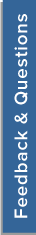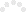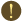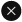We use cookies to give you the best experience on our website. If you continue to browse, then you agree to our privacy policy and cookie policy. (Last updated on: November 16, 2018).
Unfortunately, activation email could not send to your email. Please try again.# Formula not work (2)#### Replies:

19816 Oct 5,2004 12:12 AM UTC Nov 23,2004 02:45 PM UTC WinForms 3
 Subscribe to this postTags: XlsIOVu Dang Quang
Asked On October 5, 2004 12:12 AM UTC

Hi! Stephen Can you tell me how to make excel refresh value after saving without using Excel automation. For example: I''''ve file excel name is "Test.xls". In this file i have A10 range is : "=SUM(A1:A9)". I''''ve opened "Test.xls" using RWExcel component and change value of A9 and called save method. I''''ve open it again and get value of A10. But the value hasn''''t changed. If I''''ve using Microsoft Excel open it before reopen it in RWExcel component. It works ok. Thanks. vdquangReplied On October 5, 2004 09:23 AM UTC

Hi vdquang, 1) Lets assume that the value in A10 is the sum of A1:A9. A10 = SUM(A1:A9) A10 = 100 [Current Value] 2) Text.xls is opened using ExcelRW and the value of A9 is changed to 20. 3) At this point ExcelRW replaces the value of A9 but the value of A10 is still not changed since it would have to be opened using MS Excel for the calculation to take place and the value to be refreshed. 4) This is where Essential Calculate would do the job, it would calculate the value of A10 without the help of MS Excel and update the value in A10 to be 120. In other words Essential Calculate would allow you to refresh value without the need for opening the spreadsheet in MS Excel. Please let me know if you have any questions. Thanks, Stephen. >Hi! Stephen > >Can you tell me how to make excel refresh value after saving without using Excel automation. > >For example: >I''''ve file excel name is "Test.xls". In this file i have A10 range is : "=SUM(A1:A9)". >I''''ve opened "Test.xls" using RWExcel component and change value of A9 and called save method. >I''''ve open it again and get value of A10. But the value hasn''''t changed. > >If I''''ve using Microsoft Excel open it before reopen it in RWExcel component. It works ok. > > >Thanks. >vdquang >Bruce Van Horn
Replied On November 20, 2004 04:39 AM UTC

OK, so now we know we need the calculation engine, but could you complete the answer by showing us how to update the calculation using the other tool? Thanks! >Hi vdquang, > >1) Lets assume that the value in A10 is the sum of A1:A9. > >A10 = SUM(A1:A9) >A10 = 100 [Current Value] > >2) Text.xls is opened using ExcelRW and the value of A9 is changed to 20. > >3) At this point ExcelRW replaces the value of A9 but the value of A10 is still not changed since it would have to be opened using MS Excel for the calculation to take place and the value to be refreshed. > >4) This is where Essential Calculate would do the job, it would calculate the value of A10 without the help of MS Excel and update the value in A10 to be 120. In other words Essential Calculate would allow you to refresh value without the need for opening the spreadsheet in MS Excel. > >Please let me know if you have any questions. > >Thanks, > >Stephen. > >>Hi! Stephen >> >>Can you tell me how to make excel refresh value after saving without using Excel automation. >> >>For example: >>I''''ve file excel name is "Test.xls". In this file i have A10 range is : "=SUM(A1:A9)". >>I''''ve opened "Test.xls" using RWExcel component and change value of A9 and called save method. >>I''''ve open it again and get value of A10. But the value hasn''''t changed. >> >>If I''''ve using Microsoft Excel open it before reopen it in RWExcel component. It works ok. >> >> >>Thanks. >>vdquang >>Replied On November 23, 2004 02:45 PM UTC

Hi Bruce, Sorry for the delay in responding. I will post a sample shortly. Thank you for your patience. Best regards, Stephen. >OK, so now we know we need the calculation engine, but could you complete the answer by showing us how to update the calculation using the other tool? Thanks! > >>Hi vdquang, >> >>1) Lets assume that the value in A10 is the sum of A1:A9. >> >>A10 = SUM(A1:A9) >>A10 = 100 [Current Value] >> >>2) Text.xls is opened using ExcelRW and the value of A9 is changed to 20. >> >>3) At this point ExcelRW replaces the value of A9 but the value of A10 is still not changed since it would have to be opened using MS Excel for the calculation to take place and the value to be refreshed. >> >>4) This is where Essential Calculate would do the job, it would calculate the value of A10 without the help of MS Excel and update the value in A10 to be 120. In other words Essential Calculate would allow you to refresh value without the need for opening the spreadsheet in MS Excel. >> >>Please let me know if you have any questions. >> >>Thanks, >> >>Stephen. >> >>>Hi! Stephen >>> >>>Can you tell me how to make excel refresh value after saving without using Excel automation. >>> >>>For example: >>>I''''ve file excel name is "Test.xls". In this file i have A10 range is : "=SUM(A1:A9)". >>>I''''ve opened "Test.xls" using RWExcel component and change value of A9 and called save method. >>>I''''ve open it again and get value of A10. But the value hasn''''t changed. >>> >>>If I''''ve using Microsoft Excel open it before reopen it in RWExcel component. It works ok. >>> >>> >>>Thanks. >>>vdquang >>>

CONFIRMATION

This post will be permanently deleted. Are you sure you want to continue?

Sorry, An error occured while processing your request. Please try again later.You are using an outdated version of Internet Explorer that may not display all features of this and other websites. Upgrade to Internet Explorer 8 or newer for a better experience.